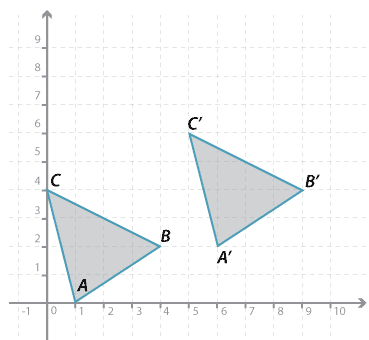#### Question 1Detailed description

$$\triangle ABC$$ is translated to $$\triangle A^\prime B^\prime C^\prime$$.

a Give the $$x$$-coordinate and the $$y$$-coordinate of:

$$x$$ = $$y$$ = Submit answer
i $$A^\prime$$ ( , ).
ii $$B^\prime$$ ( , ).
iii $$C^\prime$$ ( , ).
 b Translation to the right isThe translation is to the right and Translation up is up.

Place answers in both checkboxes before submitting.